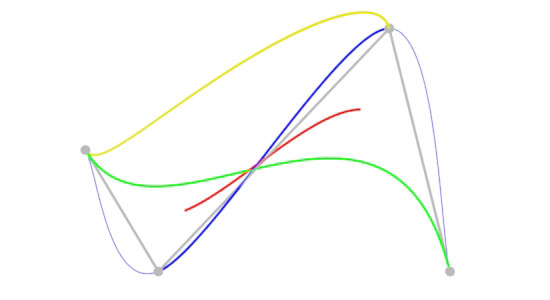### RibCurve Basics

#### Introduction

This tutorial covers the basic issues of dealing with the RenderMan's curve primitive. Figure 1 shows four colored `Curves`, that have been rendered using different "curve types" but sharing identical control vertices (cvs).

```    b-spline
bezier
catmull-rom (thick line, 4 cvs)
catmull-rom (thin line, 6 cvs - first & last cvs repeated)
hermite```

The cvs (small dots) and the lines joining them (left to right) are shown in gray.Figure 1

There is a clear difference between each of the curves. In particular, notice the green bezier curve is the only one to begin and end at the first and last cv. The RIB statements used to render the bezier curve are,

 ```Basis "bezier" 3 "bezier" 3 Bxdf "PxrConstant" "green" "color emitColor" [0 1 0] Curves "cubic"  "nonperiodic" "P" [-0.75 0 0.5 -0.45 0 1 0.5 0 0 0.75 0 1] "constantwidth" [0.01]```

 The rib file used to render this image can be viewed here curves.rib. Curves generated by Maya are of type "b-spline" ie. ` Basis "b-spline" 1 "b-spline" 1`

#### Assumptions

Although a RenderMan curve might look as if it can be defined by an arbitary number of cvs - in the example shown above the "`P`" list contains four lots of xyz's. Infact, the number of cv's must conform to formula determined by the type of curve being rendered. The simpliest curve types to use are "b-spline" and "catmull-rom" because they can be defined by any number of cvs - 4 or more cvs. Unfortunately, "b-spline" and "catmull-rom" curves not begin and end at the first and last cv. In the case of a "catmull-rom" curve if the first and last cvs are repeated then it has the desirable property of passing through all its cvs.

A "bezier" curve does have the desirable property of starting and finishing at the first and last cv. However, the number of cv's, less 1, must be exactly divisible by 3. For instance, we might use 4, 7, 10 or 13 cv's for a "bezier" curve. The only time when the restriction on the number of cv's can be ignored is when a "periodic" curve is produced, in which case, the end of the curve wraps around to coincide with the beginning of the curve. A single RenderMan `Curves` statement can define multiple separate curves.